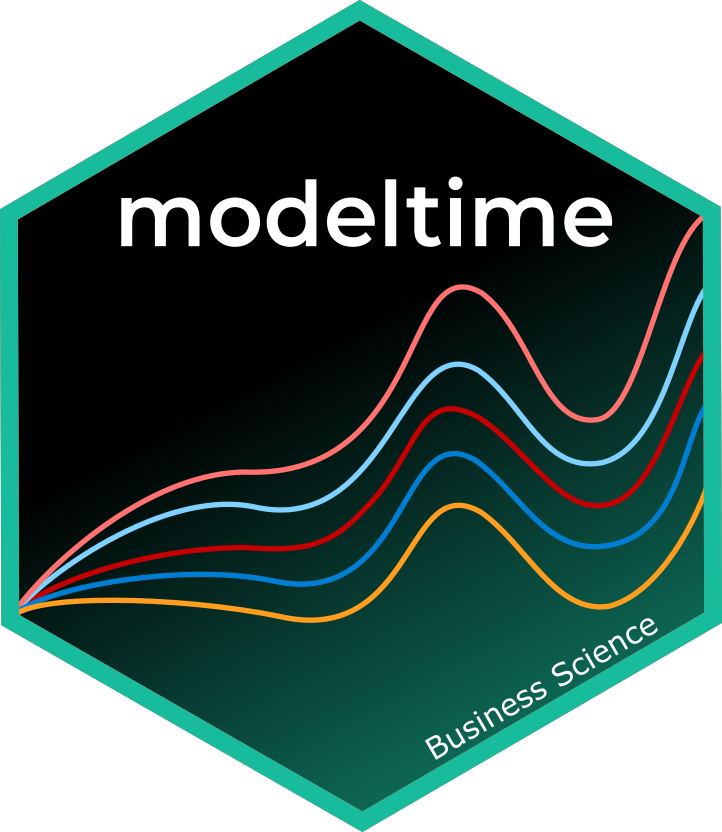Modeltime integrates Conformal Prediction Intervals as part of its time series forecasting workflow. This tutorial showcases 2 methods for Conformal Prediction Intervals:

1. Conformal Default Method: Uses qnorm() to compute quantiles from out-of-sample (test set) residuals.

2. Conformal Split Method Uses the split method split conformal inference method described by Lei et al (2018)

## Time Series Conformal Forecasting Prediction Interval Tutorial

Load libraries to complete this short tutorial.

library(tidyverse)
library(tidymodels)
library(modeltime)
library(timetk)

# This toggles plots from plotly (interactive) to ggplot (static)
interactive <- FALSE

### Step 1 - Collect data and split into training, test, and future data sets.

We’ll start with the Walmart Sales data set from timetk.

# Data

walmart_sales_tbl <- timetk::walmart_sales_weekly %>%
select(id, Date, Weekly_Sales) %>%
mutate(id = forcats::as_factor(id))

We can visualize the data set.

walmart_sales_tbl %>%
group_by(id) %>%
plot_time_series(
Date, Weekly_Sales,
.facet_ncol  = 2,
.interactive = interactive,
)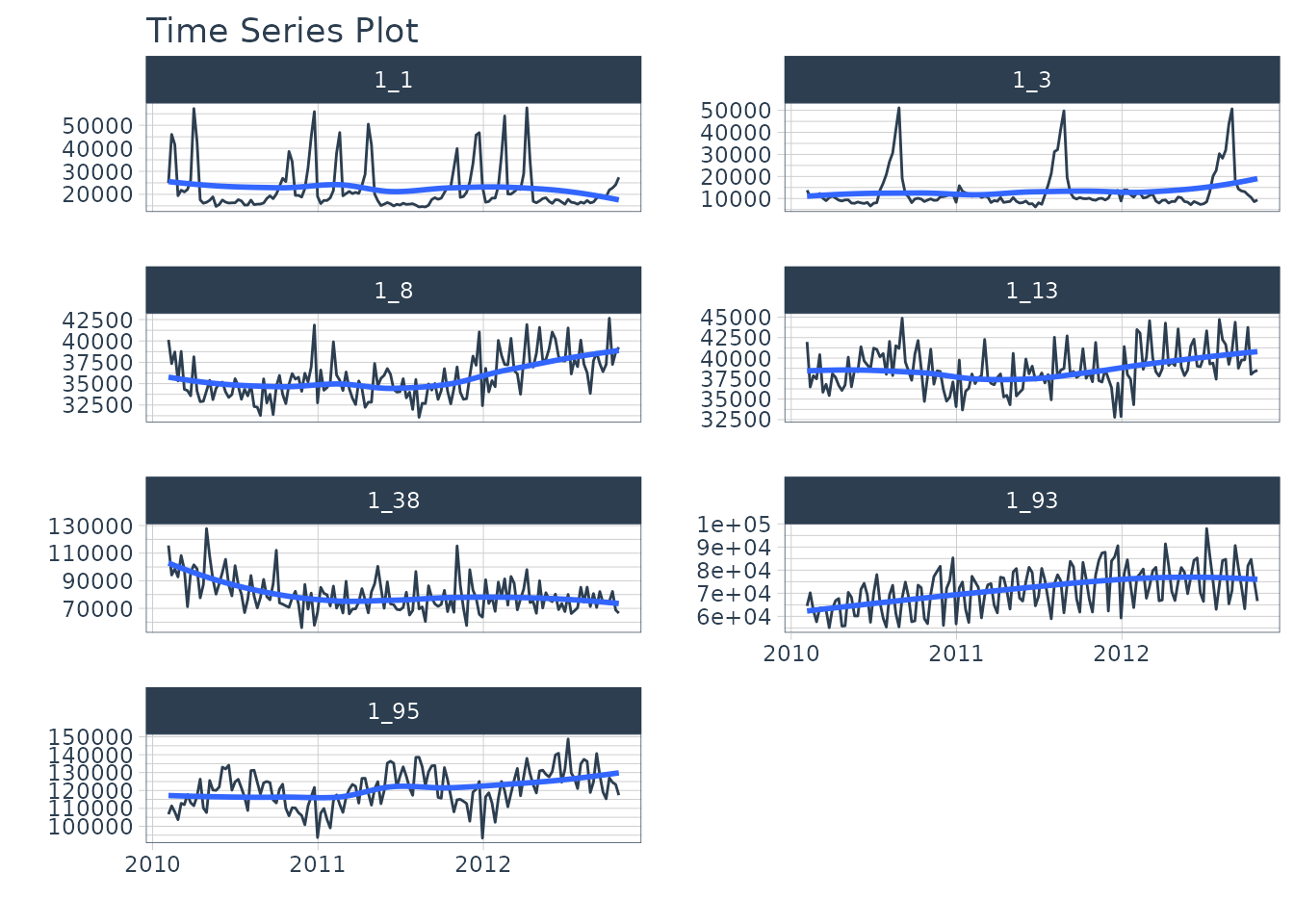Let’s split the data into training and test sets using time_series_split()

# Split Data 80/20
splits <- time_series_split(
walmart_sales_tbl,
assess     = "1 year",
cumulative = TRUE
)

splits
#> <Analysis/Assess/Total>
#> <637/364/1001>

Finally, let’s make a future dataset that will be used to forecast the next 1 year.

new_data_tbl <- walmart_sales_tbl %>%
group_by(id) %>%
future_frame(.length_out = "1 year") %>%
ungroup()

### Step 2 - Create & Fit Forecasting Models

We’ll set up an XGBoost forecasting model for this tutorial.

#### Recipe

First, let’s create a recipe. This step creates a number of time series features and one-hot encodes any categorical features.

recipe_ml <- recipe(Weekly_Sales ~ ., training(splits)) %>%
step_timeseries_signature(Date) %>%
step_rm(Date) %>%
step_dummy(all_nominal_predictors(), one_hot = TRUE)

recipe_ml

#### Model & Workflow

Next, let’s create the model and fit the recipe and model on the training dataset.

model_xgb <- boost_tree("regression") %>%
set_engine("xgboost")

wflw_fit_xgb <- workflow() %>%
fit(training(splits))

wflw_fit_xgb
#> ══ Workflow [trained] ══════════════════════════════════════════════════════════
#> Preprocessor: Recipe
#> Model: boost_tree()
#>
#> ── Preprocessor ────────────────────────────────────────────────────────────────
#> 3 Recipe Steps
#>
#> • step_timeseries_signature()
#> • step_rm()
#> • step_dummy()
#>
#> ── Model ───────────────────────────────────────────────────────────────────────
#> ##### xgb.Booster
#> raw: 44.1 Kb
#> call:
#>   xgboost::xgb.train(params = list(eta = 0.3, max_depth = 6, gamma = 0,
#>     colsample_bytree = 1, colsample_bynode = 1, min_child_weight = 1,
#>     subsample = 1), data = x$data, nrounds = 15, watchlist = x$watchlist,
#>     verbose = 0, nthread = 1, objective = "reg:squarederror")
#> params (as set within xgb.train):
#>   eta = "0.3", max_depth = "6", gamma = "0", colsample_bytree = "1", colsample_bynode = "1", min_child_weight = "1", subsample = "1", nthread = "1", objective = "reg:squarederror", validate_parameters = "TRUE"
#> xgb.attributes:
#>   niter
#> callbacks:
#>   cb.evaluation.log()
#> # of features: 3375
#> niter: 15
#> nfeatures : 3375
#> evaluation_log:
#>     iter training_rmse
#>        1     45890.002
#>        2     32737.320
#> ---
#>       14      3093.845
#>       15      2945.648

### Step 3 - Add fitted models to a Model Table.

The next step is to add model(s) to a modeltime table. This step stores the model in a data frame for organizational purposes.

models_tbl <- modeltime_table(
wflw_fit_xgb
)

models_tbl
#> # Modeltime Table
#> # A tibble: 1 × 3
#>   .model_id .model     .model_desc
#>       <int> <list>     <chr>
#> 1         1 <workflow> XGBOOST

### Step 4 - Calibrate the model to a testing set.

Next, we calibrate the model using the testing set. Note- I’m using the id = "id" which allows us to track confidence for each time series group in our dataset. The column “id” is used as the grouping column.

calibration_tbl <- models_tbl %>%
modeltime_calibrate(
new_data = testing(splits),
id       = "id"
)

calibration_tbl
#> # Modeltime Table
#> # A tibble: 1 × 5
#>   .model_id .model     .model_desc .type .calibration_data
#>       <int> <list>     <chr>       <chr> <list>
#> 1         1 <workflow> XGBOOST     Test  <tibble [364 × 5]>

## Conformal Prediction

With the calibration table in hand, we can now implement the conformal prediction interval. Currently, there are 2 methods implemented in modeltime_forecast:

1. conformal_default: Uses qnorm() to compute quantiles from out-of-sample (test set) residuals.

2. conformal_split: Uses the split method split conformal inference method described by Lei et al (2018)

### Conformal Default Method

The default method has been implemented in modeltime from the start of the modeltime package.

• This method uses qnorm() to produce a 95% confidence interval by default. It estimates a normal (Gaussian distribution) based on the out-of-sample errors (residuals).

• The confidence interval is mean-adjusted, meaning that if the mean of the residuals is non-zero, the confidence interval is adjusted to widen the interval to capture the difference in means.

Here we implement a 95% confidence interval meaning 95% of the test data will fall within the boundaries. The tail() function is used to show the .conf_lo and .conf_hi probabilistic prediction intervals.

forecast_tbl <- calibration_tbl %>%
modeltime_forecast(
new_data      = testing(splits),
actual_data   = walmart_sales_tbl,
conf_interval = 0.95,
conf_method   = "conformal_default", # Default Conformal Method
conf_by_id    = TRUE, # TRUE = local CI by ID, FALSE = global CI
keep_data     = TRUE
)

# Last 7 data points for (1 for each time series)
forecast_tbl %>% tail(7)
#> # Forecast Results
#>   # A tibble: 7 × 10
#>   .model_id .model_desc .key       .index      .value .conf_lo .conf_hi id
#>       <int> <chr>       <fct>      <date>       <dbl>    <dbl>    <dbl> <fct>
#> 1         1 XGBOOST     prediction 2012-10-26  24996.   10825.   39167. 1_1
#> 2         1 XGBOOST     prediction 2012-10-26  10884.    4842.   16927. 1_3
#> 3         1 XGBOOST     prediction 2012-10-26  33634.   25973.   41296. 1_8
#> 4         1 XGBOOST     prediction 2012-10-26  37287.   31689.   42884. 1_13
#> 5         1 XGBOOST     prediction 2012-10-26  69167.   49454.   88880. 1_38
#> 6         1 XGBOOST     prediction 2012-10-26  63422.   47315.   79529. 1_93
#> 7         1 XGBOOST     prediction 2012-10-26 111583.   95210.  127956. 1_95
#> # ℹ 2 more variables: Date <date>, Weekly_Sales <dbl>

We can visualize the probability intervals for the Conformal Default method.

forecast_tbl %>%
group_by(id) %>%
plot_modeltime_forecast(
.facet_ncol  = 2,
.interactive = interactive,
.title       = "Conformal Default"
)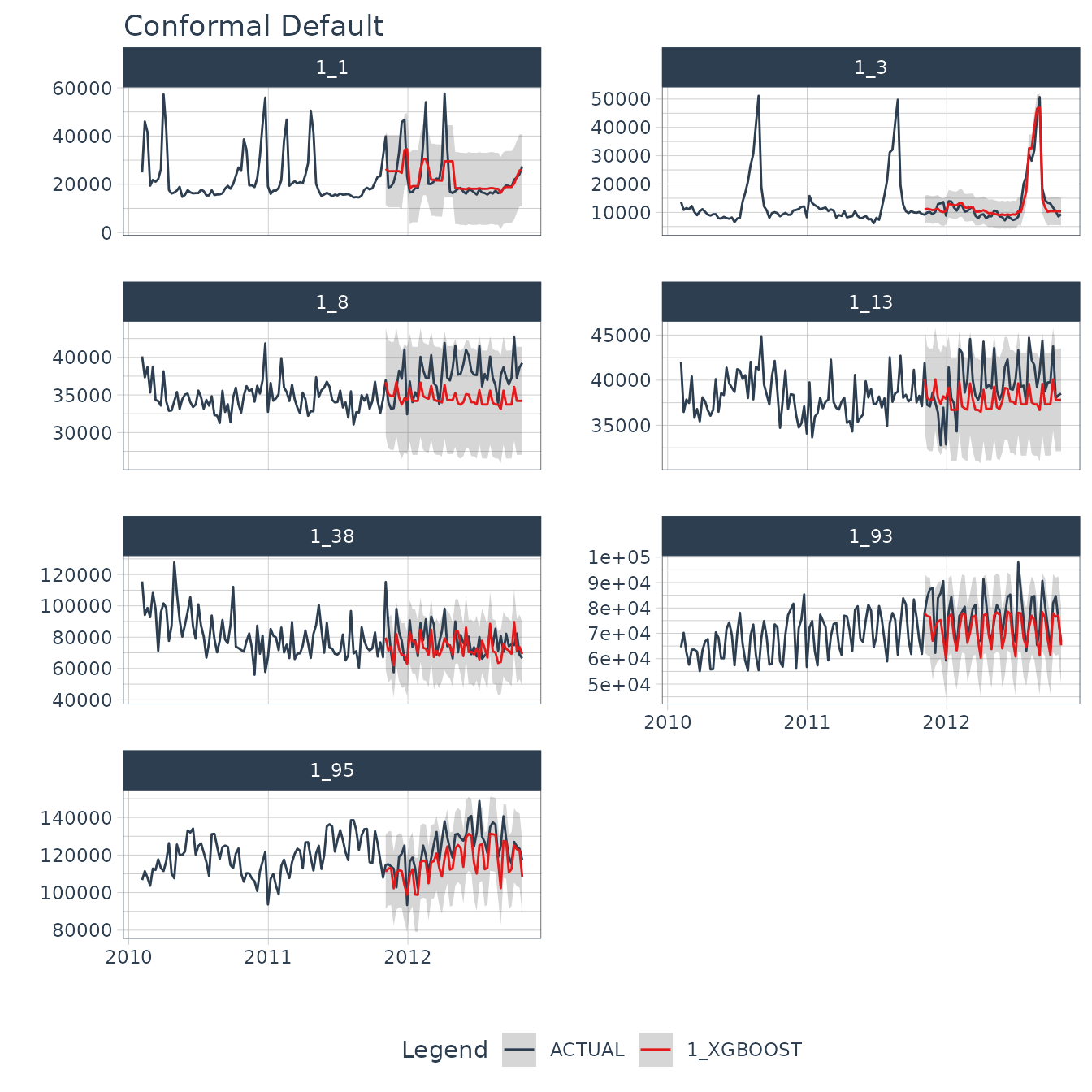### Conformal Split Method

When conf_method = "conformal_split, this method uses the split conformal inference method described by Lei et al (2018). This is also implemented in the probably R package’s int_conformal_split() function.

forecast_tbl <- calibration_tbl %>%
modeltime_forecast(
new_data      = testing(splits),
actual_data   = walmart_sales_tbl,
conf_interval = 0.95,
conf_method   = "conformal_split", # Split Conformal Method
conf_by_id    = TRUE, # TRUE = local CI by ID, FALSE = global CI
keep_data     = TRUE
)

# Last 7 data points for (1 for each time series)
forecast_tbl %>% tail(7)
#> # Forecast Results
#>   # A tibble: 7 × 10
#>   .model_id .model_desc .key       .index      .value .conf_lo .conf_hi id
#>       <int> <chr>       <fct>      <date>       <dbl>    <dbl>    <dbl> <fct>
#> 1         1 XGBOOST     prediction 2012-10-26  24996.    8291.   41701. 1_1
#> 2         1 XGBOOST     prediction 2012-10-26  10884.    3173.   18596. 1_3
#> 3         1 XGBOOST     prediction 2012-10-26  33634.   26540.   40729. 1_8
#> 4         1 XGBOOST     prediction 2012-10-26  37287.   32028.   42545. 1_13
#> 5         1 XGBOOST     prediction 2012-10-26  69167.   51069.   87265. 1_38
#> 6         1 XGBOOST     prediction 2012-10-26  63422.   46734.   80110. 1_93
#> 7         1 XGBOOST     prediction 2012-10-26 111583.   94681.  128486. 1_95
#> # ℹ 2 more variables: Date <date>, Weekly_Sales <dbl>

We can visualize the probability intervals for the Conformal Split method.

forecast_tbl %>%
group_by(id) %>%
plot_modeltime_forecast(
.facet_ncol  = 2,
.interactive = interactive,
.title       = "Conformal Split"
)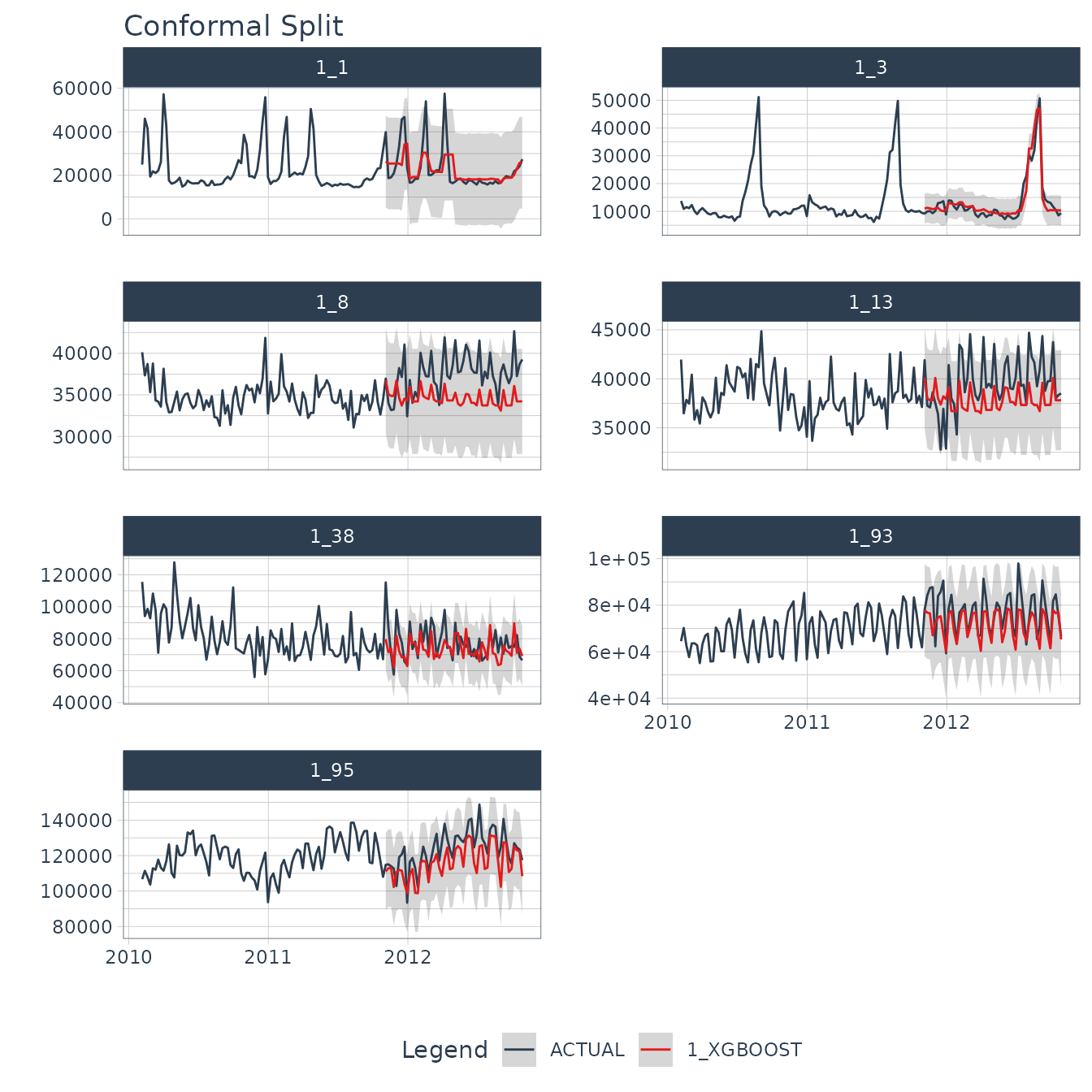## Refit and Future Forecast

Many Conformal Prediction tutorials fail to show how to make the future forecast for data that has not happened yet. I aim to fix this. Using the following code, we can quickly refit the model and make the future forecast applying the conformal probabilities to the future forecast estimates.

refit_tbl <- calibration_tbl %>%
modeltime_refit(walmart_sales_tbl)

forecast_future_tbl <- refit_tbl %>%
modeltime_forecast(
new_data      = new_data_tbl,
actual_data   = walmart_sales_tbl,
conf_interval = 0.95,
conf_method   = "conformal_split", # Split Conformal Method
conf_by_id    = TRUE, # TRUE = local CI by ID, FALSE = global CI
keep_data     = TRUE
)

With the future forecast, we can visualize both the point estimates and the 95% conformal probability region.

forecast_future_tbl %>%
group_by(id) %>%
plot_modeltime_forecast(
.facet_ncol  = 2,
.interactive = interactive,
.title       = "Conformal Split"
)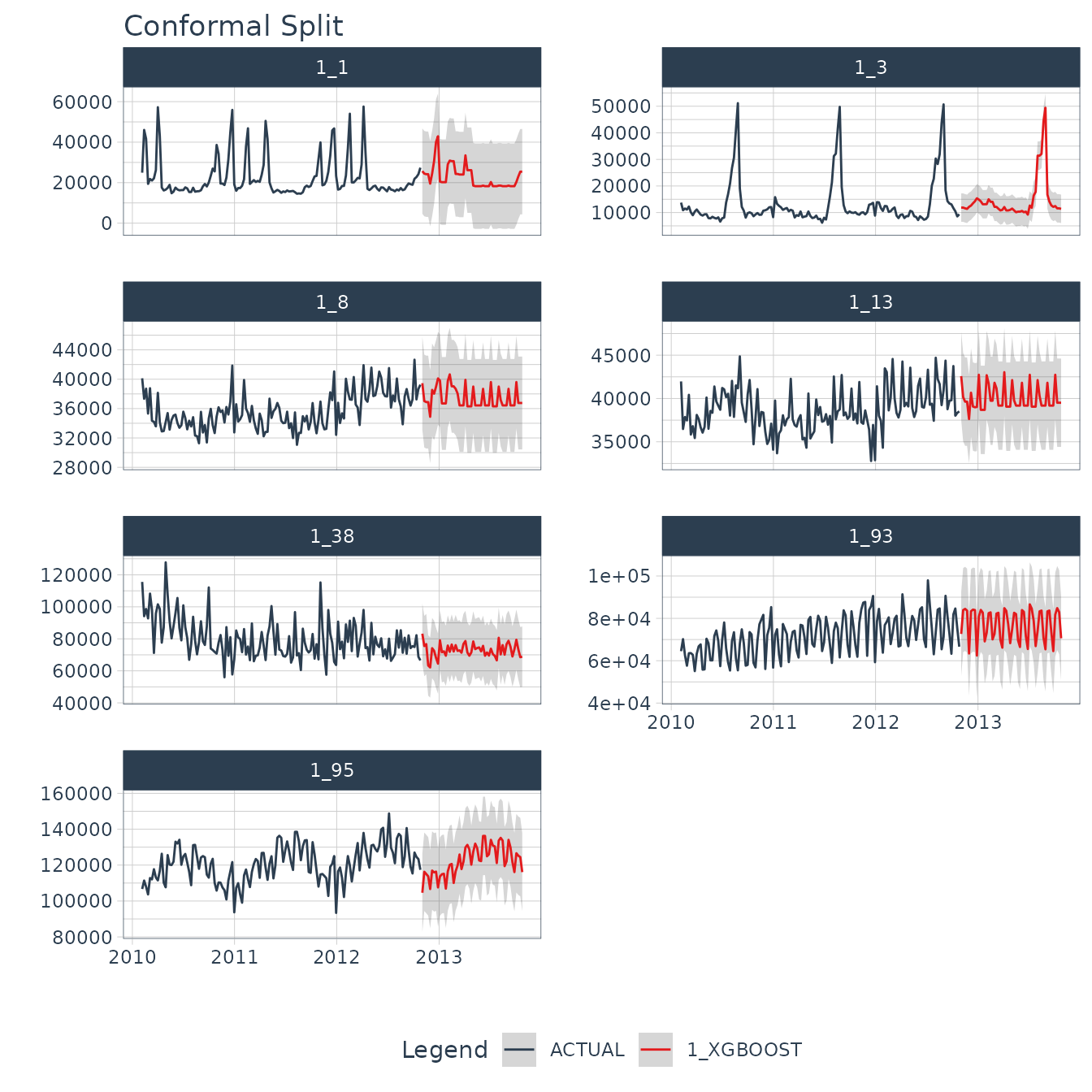## Summary

You have just seen how to do a simple Conformal Prediction estimate for a global time series model. But this is a simple problem. And, there’s a lot more to learning time series.

• Many more algorithms
• Ensembling
• Machine Learning
• Deep Learning
• Iterative Forecasting
• Scalable Modeling: 10,000+ time series

Your probably thinking how am I ever going to learn time series forecasting. Here’s the solution that will save you years of struggling.

## Take the High-Performance Forecasting Course

Become the forecasting expert for your organization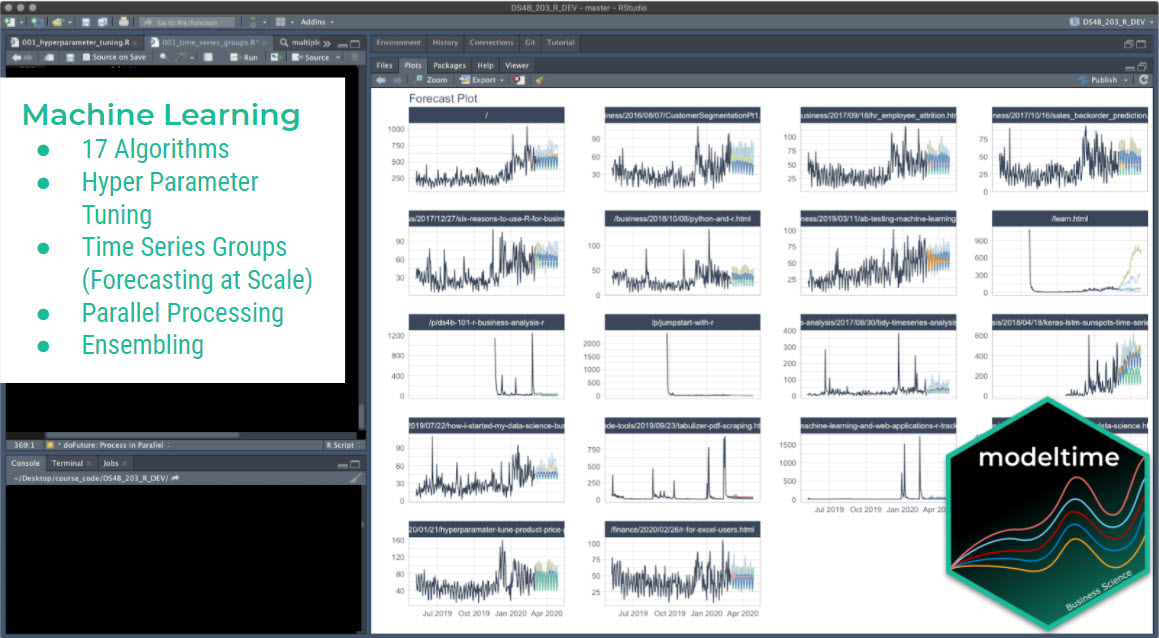High-Performance Time Series Course

### Time Series is Changing

Time series is changing. Businesses now need 10,000+ time series forecasts every day. This is what I call a High-Performance Time Series Forecasting System (HPTSF) - Accurate, Robust, and Scalable Forecasting.

High-Performance Forecasting Systems will save companies by improving accuracy and scalability. Imagine what will happen to your career if you can provide your organization a “High-Performance Time Series Forecasting System” (HPTSF System).

### How to Learn High-Performance Time Series Forecasting

I teach how to build a HPTFS System in my High-Performance Time Series Forecasting Course. You will learn:

• Time Series Machine Learning (cutting-edge) with Modeltime - 30+ Models (Prophet, ARIMA, XGBoost, Random Forest, & many more)
• Deep Learning with GluonTS (Competition Winners)
• Time Series Preprocessing, Noise Reduction, & Anomaly Detection
• Feature engineering using lagged variables & external regressors
• Hyperparameter Tuning
• Time series cross-validation
• Ensembling Multiple Machine Learning & Univariate Modeling Techniques (Competition Winner)
• Scalable Forecasting - Forecast 1000+ time series in parallel
• and more.

Become the Time Series Expert for your organization.